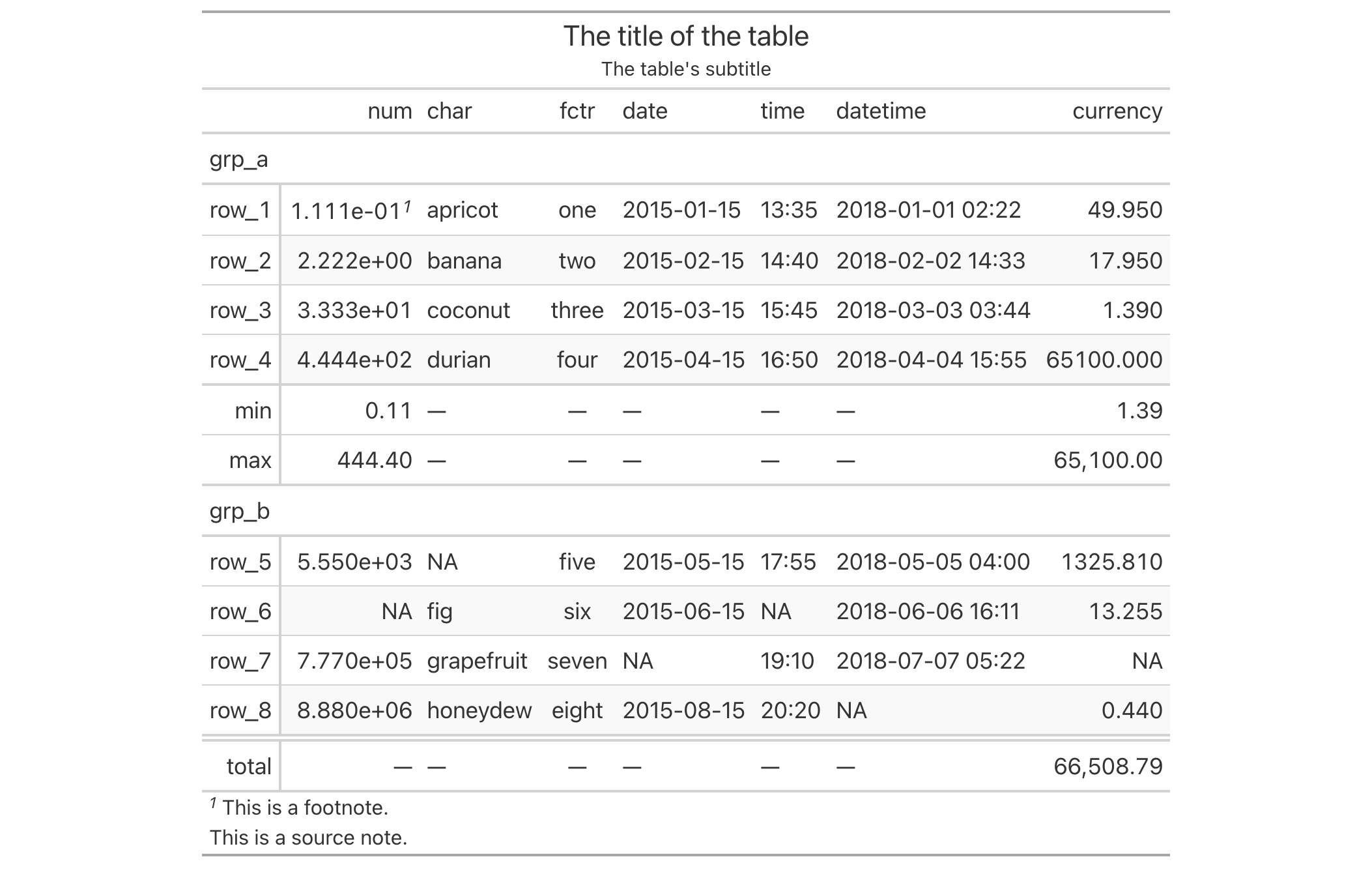# opt_row_striping: Option to add or remove row striping In gt: Easily Create Presentation-Ready Display Tables

 opt_row_striping R Documentation

## Option to add or remove row striping

### Description

By default, a gt table does not have row striping enabled. However, this function allows us to easily enable or disable striped rows in the table body. This function serves as a convenient shortcut for `<gt_tbl> %>% tab_options(row.striping.include_table_body = TRUE|FALSE)`.

### Usage

```opt_row_striping(data, row_striping = TRUE)
```

### Arguments

 `data` A table object that is created using the `gt()` function. `row_striping` A logical value to indicate whether row striping should be added or removed.

### Value

An object of class `gt_tbl`.

### Examples

Use `exibble` to create a gt table with a number of table parts added. Next, we add row striping to every second row with the `opt_row_striping()` function.

```exibble %>%
gt(rowname_col = "row", groupname_col = "group") %>%
summary_rows(
groups = "grp_a",
columns = c(num, currency),
fns = list(
min = ~min(., na.rm = TRUE),
max = ~max(., na.rm = TRUE)
)) %>%
grand_summary_rows(
columns = currency,
fns = list(
total = ~sum(., na.rm = TRUE)
)) %>%
tab_source_note(source_note = "This is a source note.") %>%
tab_footnote(
footnote = "This is a footnote.",
locations = cells_body(columns = 1, rows = 1)
) %>%
title = "The title of the table",
subtitle = "The table's subtitle"
) %>%
opt_row_striping()
```### Function ID

9-2

Other table option functions: `opt_align_table_header()`, `opt_all_caps()`, `opt_css()`, `opt_footnote_marks()`, `opt_horizontal_padding()`, `opt_stylize()`, `opt_table_font()`, `opt_table_lines()`, `opt_table_outline()`, `opt_vertical_padding()`